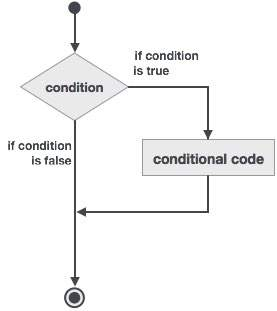# Fortran - if-then construct

An if… then statement consists of a logical expression followed by one or more statements and terminated by an end if statement.

## Syntax

The basic syntax of an if… then statement is −

```if (logical expression) then
statement
end if
```

However, you can give a name to the if block, then the syntax of the named if statement would be, like −

```[name:] if (logical expression) then
! various statements
. . .
end if [name]
```

If the logical expression evaluates to true, then the block of code inside the if…then statement will be executed. If logical expression evaluates to false, then the first set of code after the end if statement will be executed.

## Flow Diagram## Example 1

```program ifProg
implicit none
! local variable declaration
integer :: a = 10

! check the logical condition using if statement
if (a < 20 ) then

!if condition is true then print the following
print*, "a is less than 20"
end if

print*, "value of a is ", a
end program ifProg
```

When the above code is compiled and executed, it produces the following result −

```a is less than 20
value of a is 10
```

## Example 2

This example demonstrates a named if block −

```program markGradeA
implicit none
real :: marks
! assign marks
marks = 90.4
! use an if statement to give grade

gr: if (marks > 90.0) then
print *, " Grade A"
end if gr
```Grade A